Next: Priors and hyperpriors Up: No Title Previous: EM and MAP

# Construction of probabilistic models

In order to apply the Bayesian approach for modelling, the model needs to be given in probabilistic terms, which means stating the joint distribution of all the variables in the model. In principle, any joint distribution can be regarded as a model, but in practice, the joint distribution will have a simple form.

As an example, we shall see how a generative model turns into a probabilistic model. Suppose we have a model which tells how a sequence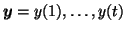transforms into sequence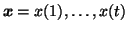.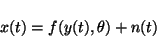(14)

This is called a generative model for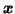because it tells explicitly how the squenceis generated from the sequence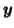through a mapping f parametrised by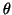. As it is usually unrealistic to assume that it would be possible to model all the things affectingexactly, the models typically include a noise term n(t).

If y(t) andare given, then x(t) has the same distribution as n(t) except that it is offset by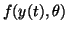. This means that if n(t) is Gaussian noise with variance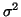, equation 14 translates into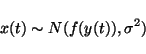(15)

which is equivalent to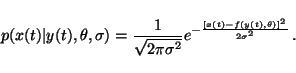(16)

The joint density of all the variables can then be written as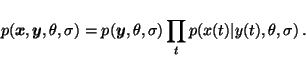(17)

Usually also the probability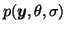is stated in a factorisable form making the full joint probability density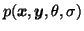a product of many simple terms.

In supervised learning, the sequenceis assumed to be fully known, also for any future data, which means that the full joint probabilityis not needed, only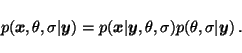(18)

Typicallyis assumed to be independent ofand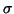, i.e.,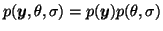. This also means thatand thus only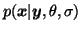, given by the generative model equation 14, and the prior for the parameters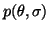is needed in supervised learning.

If the probability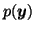is not modelled in supervised learning, it is impossible to treat missing elements of the sequence. If the probabilityis modelled, however, there are no problems. The posterior density is computed for all unknown variables, including the missing elements of. In fact, unsupervised learning can be seen as a special case where the whole sequenceis unknown. In probabilistic framework, the treatment of any missing values is possible as long as the model defines the joint density of all the variables in the model. It is, for instance, easy to treat missing elements of sequenceor mix freely between supervised and unsupervised learning depending on how large part of the sequenceis known.Next: Priors and hyperpriors Up: No Title Previous: EM and MAP
Harri Lappalainen
2000-03-03